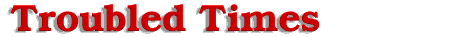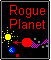#Return

As the 12th planet passes the Sun, segment 5 of the orbit begins. In segment 5, the movement of the 12th planet between the Sun and the Dark One is the same as segment 2, but reversed in direction for a total of 1,820 years. The 12th planet momentum slows due to the Sun's gravity for 4 years almost coming to a halt, and drifts again near the midpoint for 1,812 years. Once completing its stay at midpoint, the 12th planet speed increases exponentially towards the Dark One in 4 years. As the 12th planet passes the Dark One, segment 6 which is the opposite of segment 1 begins. The combined gravitational forces of the Dark One and the Sun slow forward momentum and turn the 12th planet clockwise. It is here, in segment 6 where the 12th planet has slowed enough, that the trailing gravitational wake holding the tail together gathers stray meteors, dust, and assorted other space trash, when passing through the debris fields revolving around the Dark One. Replenishing material lost during its orbital encounters with our Sun and Earth. This process continues until the carrying capacity of the tail is maximized, and the 12th planet has left the vicinity of the Dark One. Once the 12th planet reaches the furthest point of the elliptical orbit on the far side of the Dark One, the orbit is complete, and we have returned to the starting point, segment 1.

Visualizing a graphical representation of the 12th planet's movement, starting at the Dark One to the Sun where the Y axis is velocity of the 12th planet and the X axis is the passage of time, it would mimic a hyperbolic cosine curve in the shape of a wide U. A steeply decreasing hyperbolic curve, represents a rapid decrease in velocity as the 12th planet leaves the gravitational influence of the Dark One to its rear. As the Y value velocity approaches 0, the curve flattens until it is parallel to the X axis. This area of the graph would represent the time spent and the velocity of the 12th planet at the mid point. The curve stays its course essentially until the time value along the X axis reaches approximately 4 years before Earth passage. The hyperbolic curve then rapidly ascends steeply, once the 12th planet is firmly in the gravitational grasp of the Sun. The Y value, velocity, increases exponentially until the 12th planet reaches the Sun. The slope of the curve at any value T (time) equals the rate of change in velocity or acceleration. The area under the curve between 2 time intervals is equal to distance traveled by the 12th planet. The boundaries for the Distance traveled is enclosed by the hyperbolic curve at the top, the X axis at the bottom and two perpendicular lines to the X axis which represents the initial and final values of time. It is the hyperbolic function that was used to derive the distance table below.

Offered by Brent.